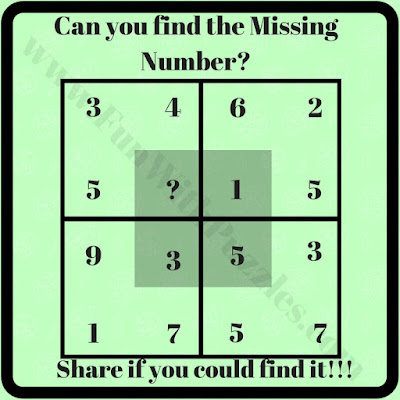Mathematical Brain Teasers can be made using different shapes. Earlier Math Brain Teasers published on this website were using different shapes like Triangle. In these Triangle Math Puzzles, one has to find the missing number in one of the triangles. Similarly, there are Circle Math Puzzles in which one has to find the missing number in one of the circles. Other than Shape Puzzles there are Finding the Odd One Out Mathematical Puzzles. Also, there are Math Puzzles in which one has to solve the given Mathematical Equations. Today's Mathematical Number Puzzles are using shapes and in these puzzles, Square Shape is used.

## How to Solve Picture Maths Number Puzzles

In these math brain teasers, there are four squares arranged in a 2x2 format. Each square contains four numbers. Numbers in each square have a certain mathematical relationship with each other. One has to find the math relationship between these numbers and has to find the missing number.

These are easy puzzles and can be solved easily by teens and adults. However, for kids, some of these puzzles can be tough. Let's see if you can find this missing number in each of the puzzles quickly? Do post your answers in the comment section of this page.1. Number Puzzle: Can you Find the Missing Number?2. Number Puzzle: Can you solve this Number Puzzle?3. Number Puzzle: Find the Missing Number4. Number Puzzle: Will you Find the Missing Number?5. Number Puzzle: Can you solve this Number Puzzle?

These Awesome Missing Number Puzzles are very much liked by kids. Solving these Maths puzzles is not only fun but will also help to enhance your brainpower. Here is the list of similar Maths Puzzles which will challenge your mind

## Easy Maths Picture Puzzles with Answers for Kids

These are easy maths pattern-finding pictures which in which your challenge is finding the missing number.

## Fun Maths Number Brain Teasers for Kids with Answers and Explanation

One more set of easy number puzzles in which your challenge is finding the common pattern of number groups around a square and then solving it for the missing number.

## Mathematics Picture Puzzles Riddles for Teens with Answers

It contains very interesting Maths Equation puzzles in which variables of the equations are replaced with symbols and your challenge is to solve the last equation.

## Tough Maths Puzzles Brain Teasers to Tickle Your Mind

Most of the puzzle links given earlier contained easy puzzles. However, this contains very tough Maths Brain Teasers which will tickle your mind.

## Answers to Awesome Missing Number Picture Maths Puzzles

To understand the explanations of these awesome missing number math squares puzzles, let's denote the number in a square with alphabets A, B, C, and D starting from the top left corner and then going clockwise.

1)

The Missing Number in the Square is 9
The logical Pattern in this puzzle is A+B = C+D

2)

The Missing Number in the Square is 10
The logical pattern linking numbers in this puzzle is that number near the center is equal to the sum of the other three numbers.

3)

The Missing Number in the Square is 4
The logical Pattern in this puzzle is A+D = C+B

4)

The Missing Number in the Square is 8
The logical Pattern in this puzzle is A+C = B+D

5)

The Missing Number in the Square is 28
The logical Pattern linking these numbers is number near the center is equal to the multiplication of the other two diagonal numbers and then subtracting the third number from the number obtained from the multiplication.

Pellarhodon said...

Square number 5 should be 28 (6*5-2 = 28). What am I doing wrong? Help me please.

Rajesh Kumar said...

You are right. Answer mentioned in the post was wrong. I have now updated the correct answer. Thanks for pointing out the correct answer for this puzzle.

Samsung said...

(3+4+5+2)-(4+3+5)=2
(6+2+3+5)-(6+2+5)=3
(6+2+1+7)-(2+1+7)=6
(5+7+3+9)-(5+7+3)=9

The solution is 9

Missing number = 9